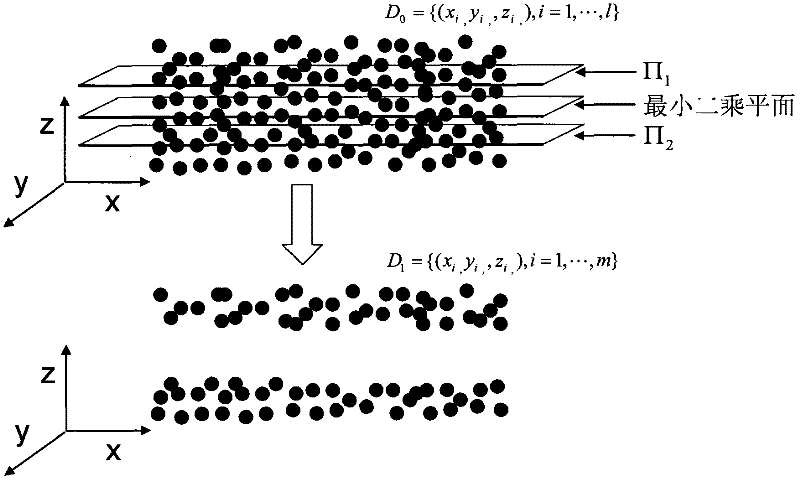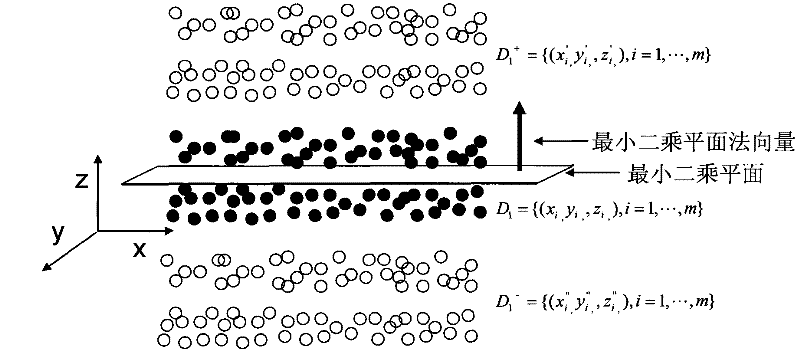# Multi-measuring-point planeness evaluation method based on support vector classification

## A technology of support vector classification and support vector machine, which is applied in the direction of measuring devices and instruments, can solve the problems of complex calculation, falling into local optimum, and difficult to guarantee the accuracy of evaluation, so as to improve calculation efficiency, improve accuracy and reduce calculation The effect of data volume

Active Publication Date: 2012-06-20
SOUTH CHINA UNIV OF TECH
2 Cites 12 Cited by

## AI-Extracted Technical Summary

### Problems solved by technology

The calculation speed of the least square method is faster, but it is difficult to guarantee the evaluation accuracy by using the least square plane instead of the minimum area plane; the calculation accuracy of the search method is high, but ...
View more

## Abstract

The invention discloses a multi-measuring-point planeness evaluation method based on support vector classification. The method comprises the following steps: sampling points on a tested surface to obtain a three-dimensional coordinate measurement value of each point; calculating a least square surface corresponding to a measurement point set; with the least square surface as a reference, removing measurement points with a small residual absolute value; moving measurement points with a big residual absolute value for the same distance respectively along the positive direction and the negative direction of the normal direction of the least square surface to form a linearly separable positive point set and negative point set; calculating the maximum interval plane of the positive point set and the negative point set with a linearly classified support vector machine method; and calculating a planeness value according to the plane. According to the multiple-measuring-point planeness evaluation method, the calculation data amount in the multiple-measuring-point planeness evaluation can be reduced and the calculation efficiency and the accuracy of an evaluation result are obviously improved.

Application Domain

Measurement devices

Technology Topic

Least squaresPositive direction +4

## Image

•••## Examples

• Experimental program(1)

### Example Embodiment

 In order to make the objectives, technical solutions and advantages of the present invention clearer, the following will further describe the embodiments of the present invention in detail with reference to the accompanying drawings:
 See figure 1 , Is a flowchart of a method for multi-point flatness evaluation based on support vector classification, the method includes the following steps:
 Step 10: Sample the points on the measured surface to obtain the three-dimensional coordinates of all the measured points.
 Step 20: Calculate the least squares plane corresponding to the measurement point set, and use the least squares plane as a reference to eliminate the measurement points with the smaller absolute value of the residual error.
 In step 30, the measurement points with large absolute values ​​are moved equally along the normal positive and negative directions of the least squares plane to form a linearly separable set of positive and negative points.
 Step 40 uses the support vector machine method of linear classification to calculate the maximum separation plane between the positive point set and the negative point set, and uses the plane to calculate the flatness value.
 See figure 2 , Is a schematic diagram of the method for removing measurement points. The method includes: Calculating the least squares plane corresponding to the measurement point set, and using the least squares plane as the reference to eliminate the measurement points with the smaller absolute value of the residual error. Measurement of the surface of the object, you can get the original measurement point set D 0 ={(x i , Y i ,,z i ,), i=1,...,l}, D 0 The equation of the corresponding least squares plane is z=Ax+By+C. According to the least squares method, the objective function is:
 minS=∑(zi-z)2=∑(zi-Ax i -By i -C) 2 (3)
 Determine the values ​​of A, B, and C by formula 1, that is, determine the position of the least squares plane; plane∏ 1 And plane∏ 2 Respectively, the distance to the least squares plane in the z-axis direction is k|e| max Two planes.
 Calculate the absolute value of the residual error in the Z-axis direction of each measurement point relative to the least squares plane |e| i :
 |e| i =|z i -Ax i -By i -C| (4)
 The maximum residual is recorded as |e| max , Remove the absolute value of the residual |e i |Less than or equal to k×|e| max (k ∈ (0, 1)) measurement point, keep the absolute value of the residual |e i |Greater than k×|e| max (k ∈ (0, 1)) measurement point, that is, the proposed plane ∏ 1 And plane∏ 2 Points between, keep the plane ∏ 1 Above and plane∏ 2 The following points, through the above operations, form a new point set D 1 ={(x i , Y i ,z i ,), i=1,...,m}. Because the measurement points that determine the minimum area plane are all located on the boundary, removing the measurement points with small residuals will not affect the evaluation results.
 See image 3 , In order to construct a schematic diagram of positive and negative points, the new measurement point set D 1 ={(x i , Y i ,z i ,),I=1,...,m} respectively move along the positive direction of the least squares plane normal vector n(A, B, -1) Form positive point set D 1 + ={(x' i , Y′ i ,,z' i ,), i=1,...,m}, move along the negative direction of the normal vector of the least squares plane Form negative point set D 1 - ={(x″ i ,Y″ i ,,z" i ,), i=1,...,m}.
 Use the support vector machine method of linear classification to analyze the above positive and negative class point set D 1 + D 1 - For classification, the specific solving process steps are as follows:
 Set the positive and negative points to D 1 + D 1 - Unified into the training set T = {(X 1 , Y 1 ), (X 2 , Y 2 ),..., (X m , Y m )}, where Xi=(x i , Y i ,z i ), Y i ={1,-1}, i=1, 2,...,m;
 Convert the problem of seeking the maximum interval into a dual problem:
 min 1 2 X i = 1 m X j = 1 m Y i Y i α i α j ( X i · X i ) - X j = 1 m α j ,
 s . t . X i = 1 m Y i α i = 0 , - - - ( 5 )
 α i ≥0, i=1, 2,..., m.
 Obtain the optimal solution through the quadratic convex programming algorithm
 Calculate the normal vector of the maximum interval plane Choose α * A positive component of And calculate the intercept of the maximum interval plane
 The maximum separation plane is (w * ·X)+b * =0, and converted to z=A 0 x+B 0 y+C 0 In this form, the plane is the measurement point set D 1 The smallest area plane, let D 1 The distance between each measuring point in the plane and the plane is d i , Then the final flatness value f MZ =d max -d min.
 The above are only preferred specific embodiments of the present invention, but the protection scope of the present invention is not limited to this. Any person skilled in the art can easily think of changes or changes within the technical scope disclosed by the present invention. All replacements shall be covered within the protection scope of the present invention. Therefore, the protection scope of the present invention should be subject to the protection scope of the claims.

## PUM## Description & Claims & Application Information

We can also present the details of the Description, Claims and Application information to help users get a comprehensive understanding of the technical details of the patent, such as background art, summary of invention, brief description of drawings, description of embodiments, and other original content. On the other hand, users can also determine the specific scope of protection of the technology through the list of claims; as well as understand the changes in the life cycle of the technology with the presentation of the patent timeline. Login to view more.

## Structure complex distribution network reliability evaluation method based on diffusion theory

Owner:SOUTHEAST UNIV

## MIMO radar waveform design method for neighboring target resolution

ActiveCN107329120AHigh resolution characteristicsImprove computing efficiency
Owner:THE PLA INFORMATION ENG UNIV

## Chirp signal parameter estimating method based on sparse constraint

Owner:XIDIAN UNIV

## Power lithium ion battery thermal runaway flue gas safety evaluation testing method

PendingCN114002385AReduce the amount of calculation datareduce workload
Owner:CRRC QINGDAO SIFANG CO LTD

## Ice coating simulation method for 220kV transmission line tower-coupling system

InactiveCN108710763AShort modeling cycleImprove computing efficiency
Owner:国网江西省电力有限公司经济技术研究院 +1

## Attendance calculating method and device

InactiveCN101702207Aavoid wasting timeImprove computing efficiency
Owner:KINGDEE SOFTWARE(CHINA) CO LTD

## Classification and recommendation of technical efficacy words

• Reduce the amount of calculation data
• Improve computing efficiency

## Power lithium ion battery thermal runaway flue gas safety evaluation testing method

PendingCN114002385AReduce the amount of calculation datareduce workload
Owner:CRRC QINGDAO SIFANG CO LTD
Who we serve
• R&D Engineer
• R&D Manager
• IP Professional
Why Eureka